# UNIT 11: Hydroxy Compounds and Ethers - Online Test

Q1. An alcohol (x) gives blue colour in Victormeyer’s test and 3.7g of X when treated with metallic sodium liberates 560 mL of hydrogen at 273 K and 1 atm pressure what will be the possible structure of X?
Explaination / Solution:

2 R - OH + 2Na 2 RONa + H22 moles of alcohol gives 1 mole of H2 which occupies 22.4L at 273K and 1 atm

number of moles of alcohol = ( 2 moles of R - OH / 22.4 L of H2 ) × 560 mL

= 0.05 moles

no. of moles = mass / molar mass

molar mass = 3.7/0.05 = 74 g mol1

General formula for R - OH Cn H2n+1 - OH

n(12) + (2n+1) (1) + 16+1=74

14n = 74 – 18

14n = 56

n = 56/14 = 4

The 2 alcohol which contains 4 carbon is CH2 CH(OH)CH2CH3

Q2. Which of the following compounds on reaction with methyl magnesium bromide will give tertiary alcohol.
Explaination / Solution: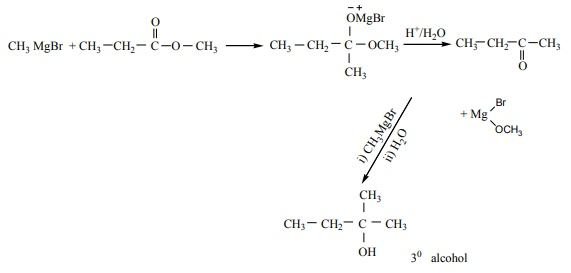Q3.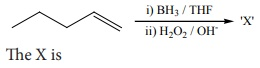Explaination / Solution:

Hydro boration – Anti markownikoft product i.e., CH3 - CH2 - CH – CH2 – CH2 - OH

Q4. In the reaction sequence, Ethene ----HOClA ---Xethan -1, 2 - diol . A and X respectively are
Explaination / Solution: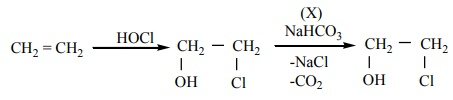Q5. Which one of the following is the strongest acid
Explaination / Solution:
No Explaination.

Q6.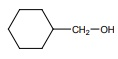on treatment with Con H2 SO4 , predominately gives
Explaination / Solution: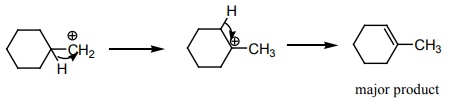Q7. Carbolic acid is
Explaination / Solution:
No Explaination.

Q8. Which one of the following will react with phenol to give salicyladehyde after hydrolysis.
Explaination / Solution:

Riemer – Tiemann reaction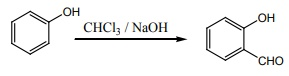Q9. (CH3 )3 - C - CH(OH) CH3 -----Con H2 SO4X (major product)
Explaination / Solution: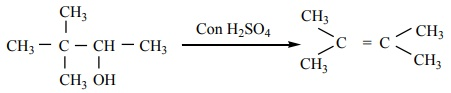Q10. The correct IUPAC name of the compound,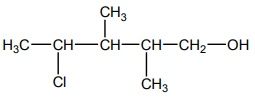Explaination / Solution:
No Explaination.